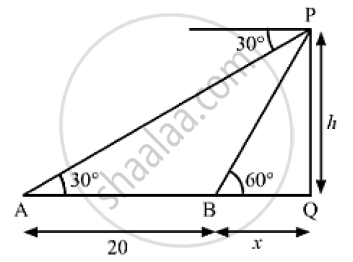# The Angle of Depression Form the Top of a Tower of a Point a on the Ground is 30° , Find the Height of the Tower and Its Distance from the Point A. - Mathematics

The angle of depression form the top of a tower of a point A on the ground is 30° . On moving a distance of 20 meters from the point A towards the foot of the tower to a point B, the angle of elevation of the top of the tower to from the point B is 60° . Find the height of the tower and its distance from the point A.

#### SolutionLet PQ be the tower.
We have,
AB = 20m, ∠PAQ = 30°  and ∠PBQ = 60°
Let BQ = x and PQ =  h
In  ΔPBQ,

 tan 60° = (PQ)/(BQ)

⇒ sqrt(3) = h/x

⇒ = x sqrt(3)                .......(1)

Also, in ΔAPQ ,

 tan 30° = (PQ)/(AQ)

⇒ 1/ sqrt(3) = h/(AB +BQ)

⇒ 1/ sqrt(3) = (x sqrt(3))/(20+x)                      [Using (1)]

⇒ 20+ x = 3x

⇒ 3x -x = 20

⇒ 2x = 20

⇒ =20/2

⇒ x = 10 m

From (i),
h = 10 sqrt(3)=10xx1.732 =17.32m
Also, AQ = AB+BQ = 20 + 10 = 30m
So, the height of the tower is 17. 32 m and its distance from the point A is 30 m.

Concept: Heights and Distances
Is there an error in this question or solution?
Chapter 14: Height and Distance - Exercises

#### APPEARS IN

RS Aggarwal Secondary School Class 10 Maths
Chapter 14 Height and Distance
Exercises | Q 20

Share